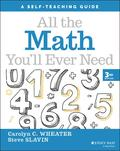John Wiley & Sons All the Math You'll Ever NeedA comprehensive and hands-on guide to crucial math concepts and terminology In the newly revised th.. Product #: 978-1-119-71918-2 Regular price: \$21.40 \$21.40 Auf Lager

# All the Math You'll Ever Need

## A Self-Teaching Guide

Wiley Self-Teaching Guides3. Auflage April 2022
336 Seiten, Softcover
Wiley & Sons Ltd

ISBN: 978-1-119-71918-2
John Wiley & Sons

Jetzt kaufen

### Preis: 22,90 €

Preis inkl. MwSt, zzgl. Versand

A comprehensive and hands-on guide to crucial math concepts and terminology

In the newly revised third edition of All the Math You'll Ever Need: A Self-Teaching Guide, veteran math and computer technology teacher Carolyn Wheater and veteran mathematics author Steve Slavin deliver a practical and accessible guide to math you can use every day and apply to a wide variety of life tasks. From calculating monthly mortgage payments to the time you'll need to pay off a credit card, this book walks you through the steps to understanding basic math concepts.

This latest edition is updated to reflect recent changes in interest rates, prices, and wages, and incorporates information on the intelligent and efficient use of calculators and mental math techniques. It also offers:
* A brand-new chapter on hands-on statistics to help readers understand common graphs
* An easy-to-use-format that provides an interactive method with frequent questions, problems, and self-tests
* Complete explanations of necessary mathematical concepts that explore not just how math works, but also why it works

Perfect for anyone seeking to make practical use of essential math concepts and strategies in their day-to-day life, All the Math You'll Ever Need is an invaluable addition to the libraries of students who want a bit of extra help applying math in the real world.

How to Use This Book vii

Acknowledgments

1 Getting Started

2 Essential Arithmetic

What Is Multiplication?,

Subtraction

Division

3 Focus on Multiplication

The Multiplication Table,

Learning the Multiplication Table,

Practice Exercises with Two Digits,

Multiplying Three Digits,

Four-Digit Multiplication,

Word Problems with Multiplication,

4 Focus on Division

Short Division,

Long Division,

Word Problems with Division,

5 Mental Math

Fast Multiplication,

Fast Division,

How Are You Doing So Far?,

Mental Math Tricks

6 Positive and Negative Numbers

What Are Negative Numbers?,

Subtracting Positive and Negative Numbers,

Multiplying Positive and Negative Numbers,

Division with Positive and Negative Numbers,

7 Fractions

Changing the Look of Fractions,

Subtracting Fractions,

Multiplying by Fractions,

Dividing by Fractions,

8 Decimals

Multiplying with Decimals,

Dividing with Decimals,

9 Converting Fractions and Decimals

Converting Fractions into Decimals,

Converting Decimals into Fractions,

Simplifying Fractions,

Repeating Decimals

Non-terminating Decimals

10 Ratios and Proportions

Ratios,

Proportions,

11 Solving Problems

Words and Fractions,

Word Problems with Money,

12 Area and Perimeter

Areas of Rectangles,

When We Use Area,

Perimeters of Rectangles,

Areas of Triangles,

13 Circumference and Area

Circumferences of Circles,

The Area of a Circle,

14 Percentages

Fractions, Decimals, and Percents,

Percent Change,

Fast Percent Change,

Percent Distribution,

Tipping,

15 Solving Simple Equations

The Care and Treatment of Equations,

Isolating x,

Multiplication and Division with x,

Fractions and Decimals in Equations,

Negative Numbers,

Multi-Step Equations,

16 Powers and Roots

Exponents,

Roots,

17 Very Large and Very Small Numbers

Writing Large Numbers,

Scientific Notation,

Millions, Billions, Trillions, and Beyond

Multiplying Large Numbers,

Dividing Large Numbers,

Scientific Notation for Very Small Numbers,

18 Algebra Problems

Age Problems,

Finding the Numbers,

Mixture Problems,

19 Interest Rates

Simple Interest,

Compound Interest,

A Word on Continuous Compounding,

Doubling Time: The Rule of 70,

Discounting,

20 Rate, Time and Distance

The Magic Formula,

The Terms,

Finding Distance,

Finding Rate,

Finding Time,

Rate, Time, and Distance Problems,

Speed Limit Problems,

21 Personal Finances

Mark-Down Problems,

Sales Tax Problems,

Credit Cards,

Federal Income Tax,

Mortgage Interest and Taxes,

Commissions,

Mark-Ups,

Discounting from List Price,

Quantity Discounts,

2/10 n/30,

Chain Discounts,

Profit

23 A Taste of Statistics

Distributions of Data,

Measures of Center,